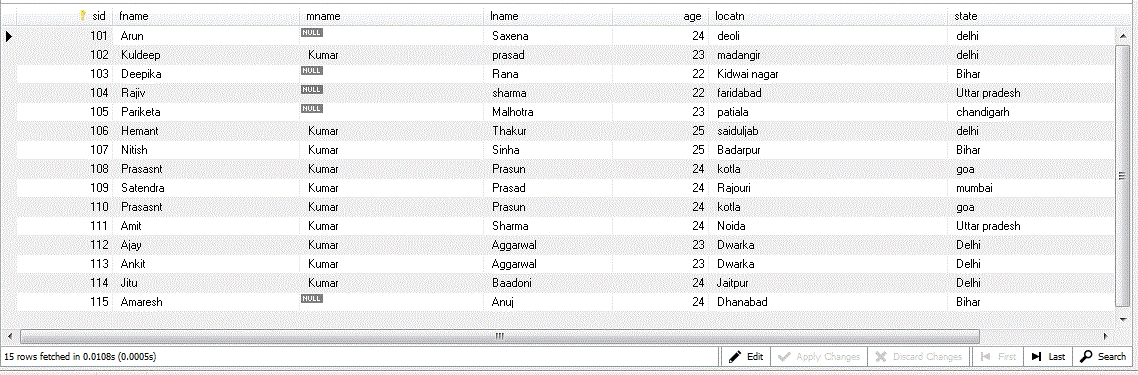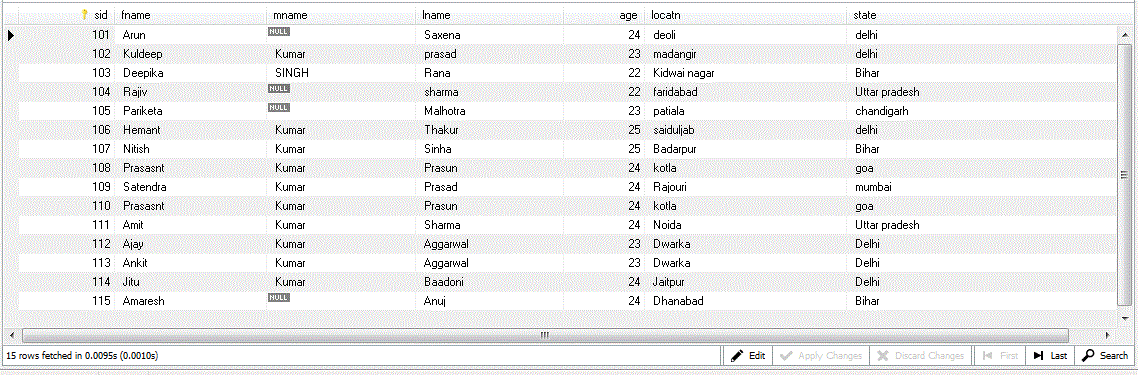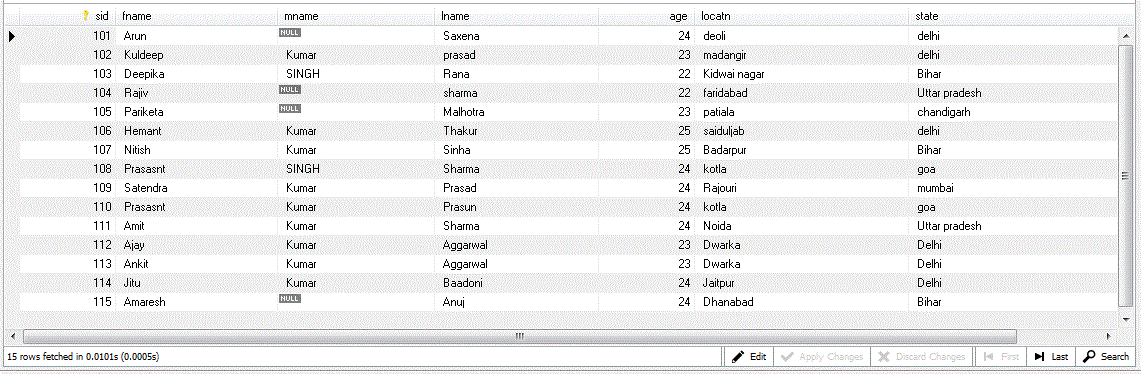MySQL

Update in MySQL
 Previous Home Next

The update command is use to modify the stored data or existing records in the table. the are some clause which use in this command like:

1. The update can be done in one or more field.
2. In this we can also use the WHERE condition.

Note: The updation can be done single table at a time.

Syntax

```UPDATE tablename
SET field1 = new-value1, field2=new-value2
[WHERE Clause]
```

Previous view of table is shown belowExample

```UPDATE studinfo 				//Update command
SET mname='SINGH' 				//insert value
WHERE sid=103;					//Where conditions
```Update one or more values in a same table

Example

```UPDATE STUDINFO					//update command
SET mname=NULL ,mname='SINGH'			//More than one condition
WHERE SID=108 and 105;				//where condition
```Previous Home Next